# Moving functions

Moving formulas can be used to smooth out any irregularities in your data to easily recognize trends. The larger the interval you set, the more the peaks and valleys are smoothed out. While the smaller the interval, the closer the moving averages are to the actual data points.

Each of the moving formula accepts a measure, two integers to define the window, and one or more optional attributes.

`formula (measure,integer,integer,[attribute,attribute,...])`

Only the measure and integer values are required. If you supply both required and optional values, the formula returns the aggregate of the measure over the given window. You should experiment with only a measure and integers, leaving out the attribute, and then adding it back in. This will help you decide which output best meets your use case.

The time window is (`current - Num1...Current + Num2`), including both endpoints. For example, `1,1` has a window size of 3. To see periods in the past, use a negative number for the second endpoint, as in the example `moving_average(sales, 1, -1, date)`.

For more information on how the time windows work, see this chart: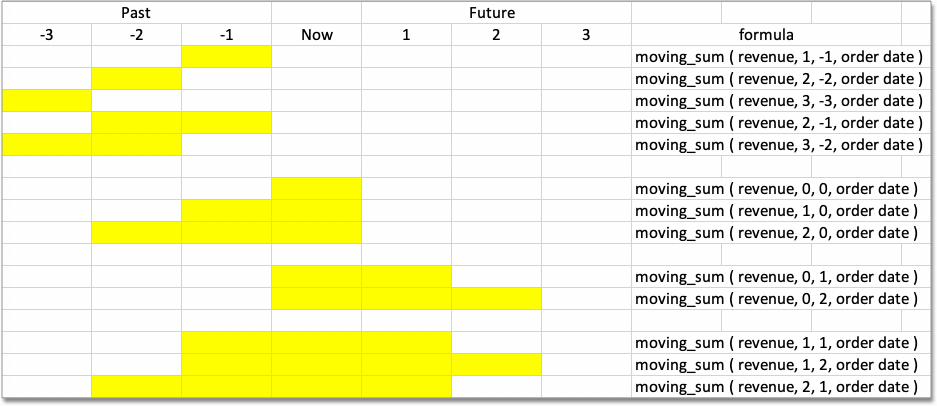The moving formulas are the following:

• `moving_average`, for example `moving_average (revenue, 2, 1, customer region)`

Takes a measure, two integers to define the window to aggregate over, and one or more attributes. Returns the average of the measure over the given window. The attributes are the ordering columns used to compute the moving average.

• `moving_max`, for example `moving_max (complaints, 1, 2, store name)`

Takes a measure, two integers to define the window to aggregate over, and one or more attributes. Returns the maximum of the measure over the given window. The attributes are the ordering columns used to compute the moving maximum.

• `moving_min`, for example `moving_min (defects, 3, 1, product)`

Takes a measure, two integers to define the window to aggregate over, and one or more attributes. Returns the minimum of the measure over the given window. The attributes are the ordering columns used to compute the moving minimum.

• `moving_sum`, for example `moving_sum (revenue, 1, 1, order date)`

Takes a measure, two integers to define the window to aggregate over, and one or more attributes. Returns the sum of the measure over the given window. The attributes are the ordering columns used to compute the moving sum.

## Calculate a moving average

This example demonstrates using the `moving_average` formula. To use the moving function in a search:

1. Start a new search, or edit an existing Answer.

2. Open the Data panel from the upper-right corner if it is not open, and select the + icon next to Formulas. If the new Answer experience is off in your environment, select the More options icon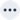in the upper-right side of the table, and select Add formula.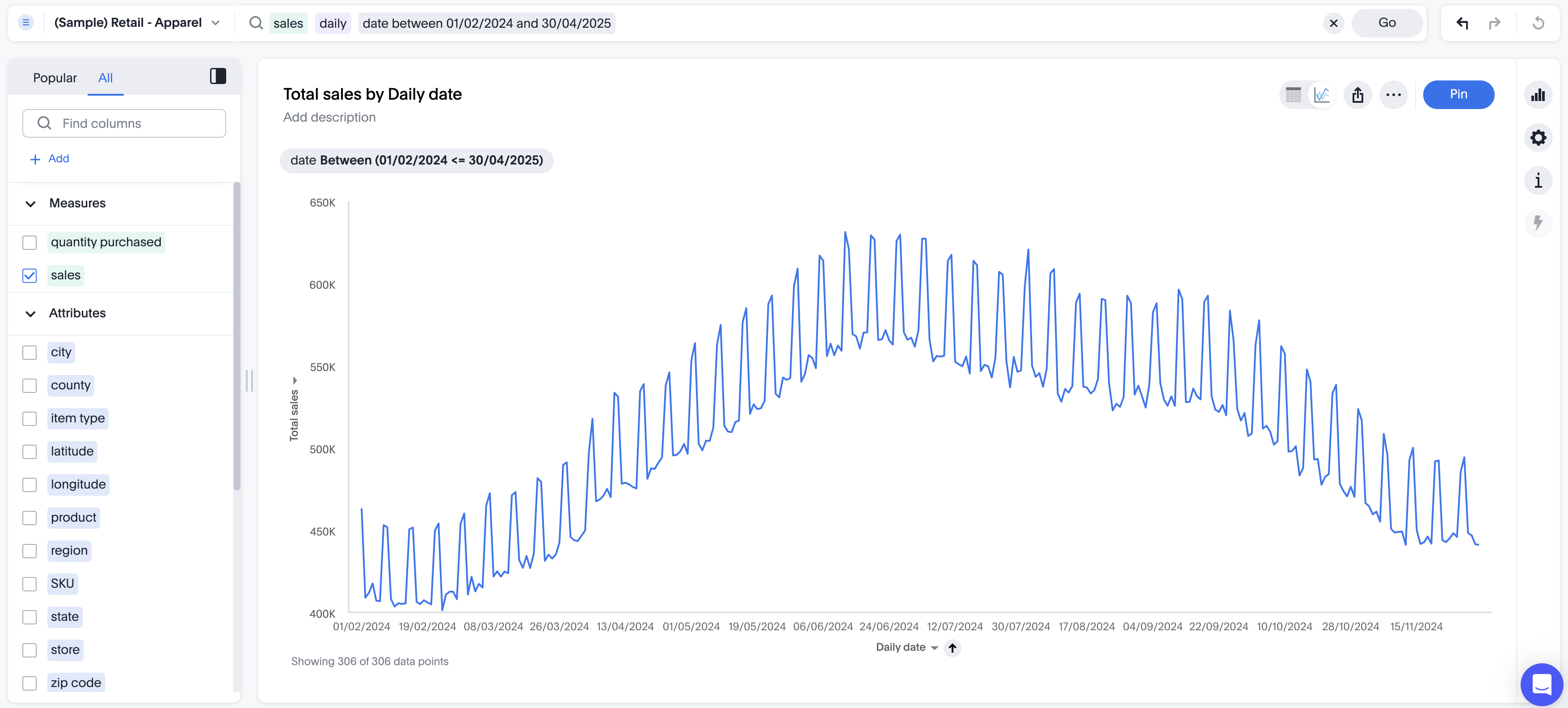3. Enter the moving_average formula, providing a measure, a window, and one or more attributes.

The example returns the average of revenue, within the commit date window size of 3. The window includes the previous, current, and next rows. The attributes are the ordering columns used to compute the moving average. The window is (current - Num1…​Current + Num2) with both end points being included in the window. For example, "1,1" will have a window size of 3. To see periods in the past, use a negative number for the second endpoint, as in the example "moving_average(revenue, 1, -1, date)".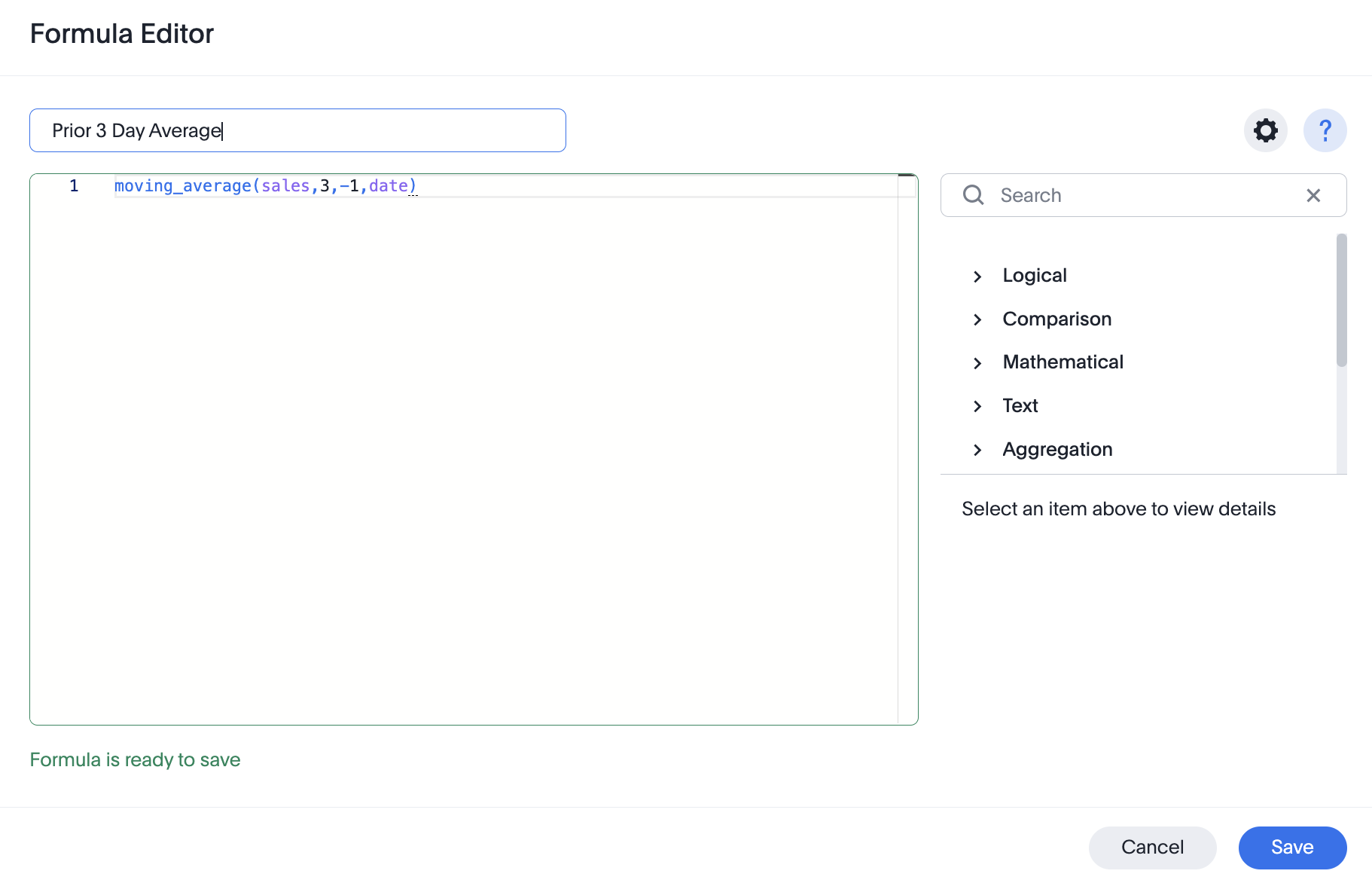4. Name the formula by entering a title in the top field, and then select Save.

The formula appears in the search bar and in the table as its own column.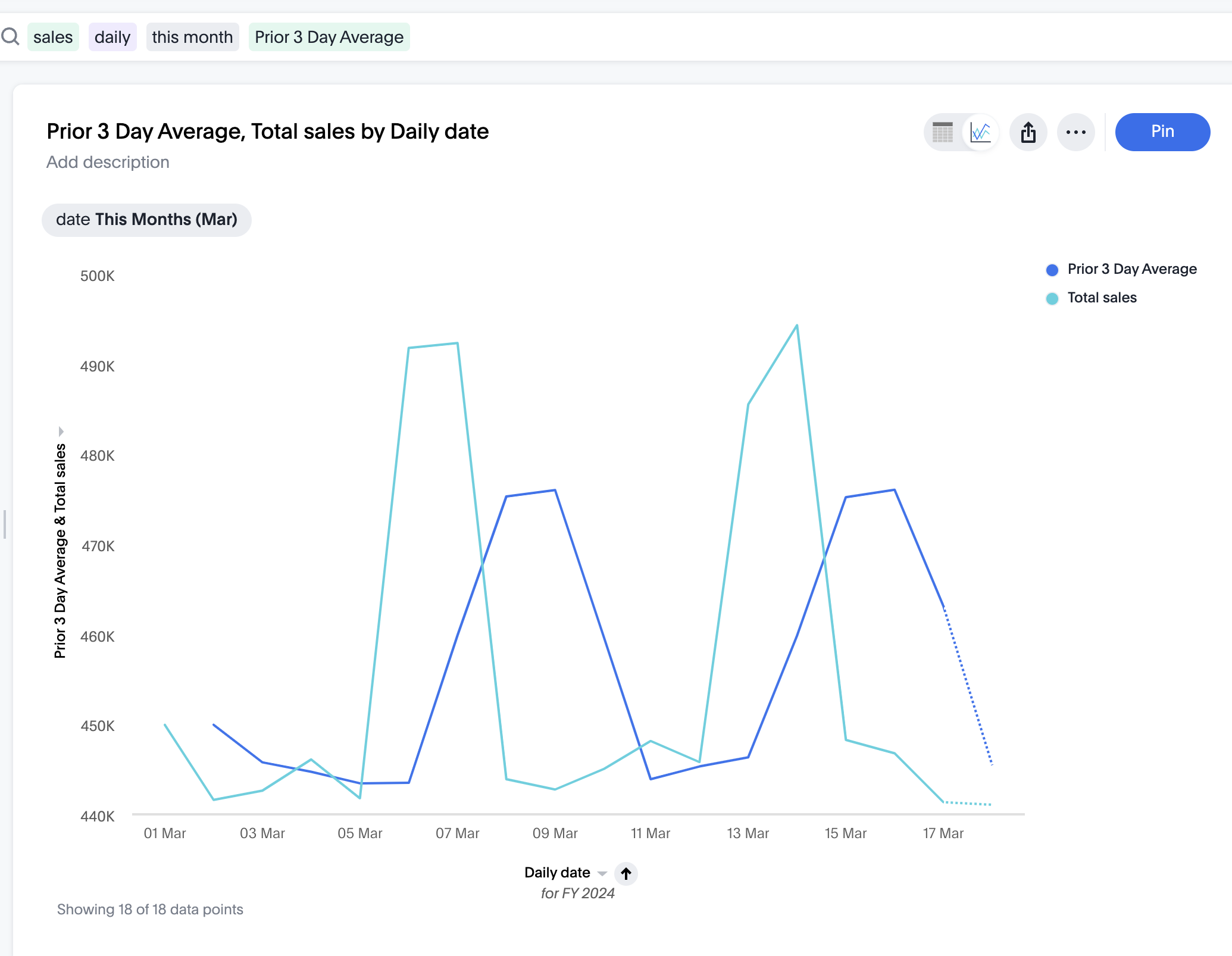A box displaying the moving average within the entire table will appear at the bottom.

5. To use a different aggregation type, click the current aggregation type in the bottom of the box and select another type.

## Calculate a moving sum of a unique count

ThoughtSpot doesn’t have a purpose-built function for calculating a moving sum, or other moving formula, for a unique count. However, for many use cases, you can use a `group_aggregate` to facilitate this type of count. Adding a `group_aggregate` to your formula allows you to pass the aggregate function (in this case, `unique count`) in the windowing function (in this case, `moving_sum`).

See the following example for the general formula for a moving sum of a unique count. In this example, we calculate the moving sum of unique customer names, using `monthly date` as our aggregation bucket.

``moving_sum (group_aggregate (unique count (Customer Name ),query_groups (),query_filters ()),2,0,start_of_month (Date ))``

The result looks something like this: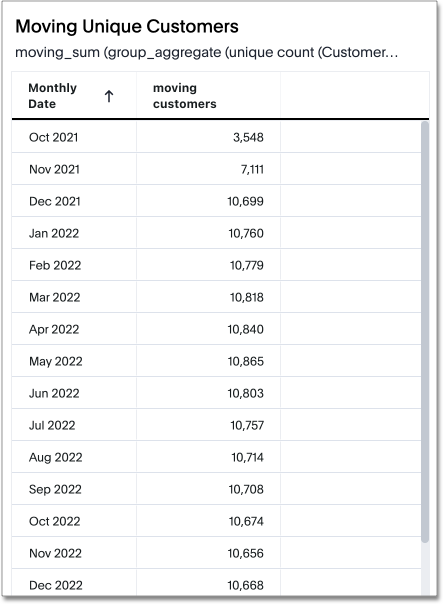You can use this syntax on any moving formula: average, maximum, minimum, and sum.

 This syntax produces a unique count for each individual result, such as Oct 2021. However, it doesn’t produce a unique count across results. If a customer name appears in both October 2021 and November 2021, for example, that customer would be counted in both those months.